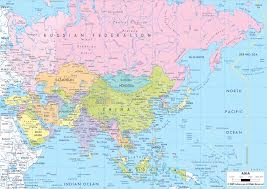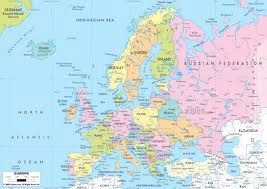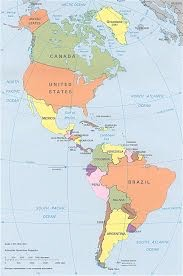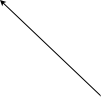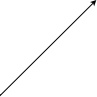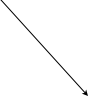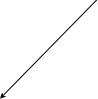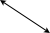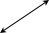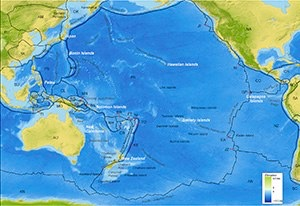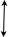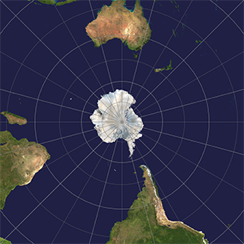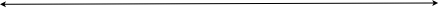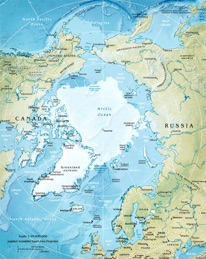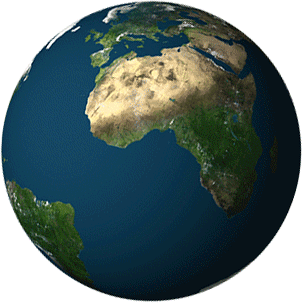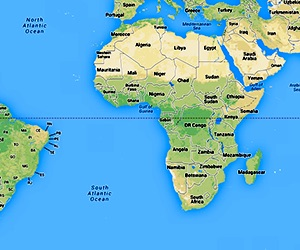A manifold is an abstraction which generalizes the concept of embedded surface in R^3 and is the basic object studied in differential geometry. The underlying idea is similar to how cartographers describe the earth: there is a map, i.e., a plane representation, for every part of Earth and if two maps represent the same location or have an overlap, there is a unique (smooth) way to identify the overlapping points on both maps. Similarly, a manifold should look locally like R^n, i.e. there are maps which identify parts of the manifold with the flat space R^n and if two maps describe overlapping regions, there is a unique smooth way to identify the overlapping points. Most of the notions from calculus on R^n are local in nature and hence can be transported to manifolds. Further, some nonlocal constructions, such as integration, can be performed on manifolds using patching arguments.

This course will cover the following concepts:

1. definition and examples of manifolds

2. quotients and Lie groups,

3. tangent and cotangent spaces as well as vector bundles,

4. vector fields and forms, as well as sections of vector bundles,

5. submanifolds,

6. diffeomorphisms,

7. distributions,

8. tensor and exterior algebras,

9. exterior derivative and de Rham cohomology,

10. integration and Stoke’s theorem.

The course will also cover the following important results relating the concepts above:

1. implicit and inverse function theorems,

2. Cartan calculus,

3. Frobenius theorem,

4. Stoke’s theorem.

Material covered so far:

Lee’s chapters: 1, 2, 3, 4, 5, 8 (except for the Lie algebra of a Lie group), 9, 10 and 11 (except line integrals and conservative vector fields), 12, 14, 15, 16 (except for the lat two sections), 17 (first section only) and the notes on Cech cohomology.

Thursday 6 November 2014 (Week 45)

The second exam will take place. See Osiris for up to date information

Thursday 30 October 2014 (Week 44)

This lecture we studied integration and Stokes theorem.

Tuesday 28 October 2014 (Week 44)

This lecture we introduced the general Lie derivatives of tensors, orientations and manifolds with boundary.

Thursday 23 October 2014 (Week 43)

This lecture we introduced tensor fields on manifolds and introduced the exterior derivative.

Tuesday 21 October 2014 (Week 43)

This lecture we continued our study of tensors.

Thursday 16 October 2014 (Week 42)

This lecture we introduced tensors.

Tuesday, 14 October 2014 (Week 42)

Exam 1. HFG 611 from 9:00.

Thursday 9 October 2014 (Week 41)

This lecture we studied the question of extension of functions defined on submanifolds. We then moved on to the study of flows of vector fields.

Tuesday 7 October 2014 (Week 41)

This lecture we studied of the inverse function theorem and related results. The exam contains only material covered up to and inclusive this lecture.

Thursday 2 October 2014 (Week 40)

This lecture we studied further the cotangent bundle. We defined the exterior derivative of functions as well as the pull back of functions and forms. We defined the Lie bracket of vector fields and studied its basic properties. We then moved on to the study of submanifolds.

Tuesday 30 September 2014 (Week 40)

This lecture we continued our study of vector bundles. We proved the chart lemma and introduced the pull back of vector bundles. We saw how linear algebraic constructions can be applied fiberwise to bundles to produce new bundles. We studied maps between bundles and finally introduced the cotangent bundle of a manifold.

Thursday 25 September 2014 (Week 39)

This lecture we continued our study of the tangent bundle and introduced the more general notion of vector bundles.

Tuesday 23 September 2014 (Week 39)

This lecture we introduced tangent vectors and the tangent bundle of a manifold.

Thursday 18 September 2014 (Week 38)

This lecture we continued our study of Cech cohomology (now with other coefficients). We proved that Cech cohomology with coefficients on smooth functions in only nontrivial in degree 0 where it agrees with globally defined functions.

Tuesday 16 September 2014 (Week 38)

This lecture we studied covers, subcovers, refinements, partitions of unity and introduced Cech cohomology with real coefficients.

Thursday 11 September 2014 (Week 37)

This lecture we took a second look at second countability, saw several examples of manifolds (R^n, vector spaces, open subsets of manifolds, the general linear group, spheres and real and complex projective spaces). In particular we saw that CP^1 and S^2 are diffeomorphic. Finally we proved a “cover lemma” for manifolds, namely, that one can construct a manifold out of open sets of R^n together with smooth “change of coordinates” which satisfy a skew symmetry and a “cocycle condition”.

Tuesday 9 September 2014 (Week 37)

This lecture we covered the definition and examples of topological and smooth manifolds and introduced the notion of smooth map between manifolds.Differential geometry

Homework and Hand-in assignments

Exercise sheet 14

Announcements

All marks are out

Practical information

Lectures:

Tuesdays from 9:00 to 11:00 at room 611 in the maths building;

Thursdays from 13:15 to 15:00 at MIN 027.

Exercise classes:

Tuesdays from 11:00 to 13:00 at room 611 in the maths building;

Thursdays from 15:00 to 17:00 at MIN 012.

The book we will be using as reference for this course is Lee’s “Introduction to smooth manifolds”. A legal pdf copy of the book can be obtained from the UU library  by following the springer link and searching for the title.

Other references you can use include:

1. Lang, S. Fundamentals of differential geometry. Graduate texts in mathematics. Springer Verlag.

2. Looijenga, E. Lecture notes on Smooth manifolds.

3. Spivak, M. A comprehensive introduction to Differential geometry, vol 1. Publish or Perish.

4. Spivak, M. Calculus on manifolds. Addison--Wesley Publishing company.

5. Warner, F. Foundations of Differentiable manifolds and Lie groups. Graduate texts in mathematics 94. Springer Verlag.

There will be regular hand-in exercises and two exams for this course, the first on 14/Oct (week 42) and the second on 4/Nov (week 45).

The the hand-in exercises contribute with 20% of the final mark, the first exam contributes with 30% and the last with 50%. If you do the re-take exam, the hand-in exercises contribute with 20% of the final mark and the retake with 80%.